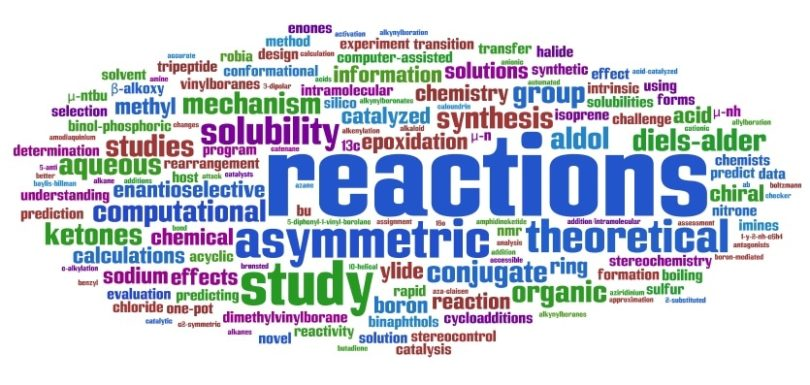# Redox Reactions Questions### Questions on Oxidation-Reduction Reactions (Redox Reactions):

Ques. The compound YBa2Cu3O7 which shows superconductivity has copper in oxidation state …….. Assume that the rare earth element Yttrium is in its usual +3 oxidation state
(a) 3/7
(b) 7/3
(c) 3
(d) 7

Ans. (b)

Ques. The oxidation state of I in H4IO6 is
(a) + 7
(b) + 5
(c) + 1
(d) – 1

Ans. (a)

Ques. The oxidation number of hydrogen in LiH is
(a) + 1
(b) – 1
(c) 2
(d) 0

Ans. (b)

Ques. The number of moles of KMnO4 reduced by one mole of KI  in alkaline medium is:
(a) One fifth
(b) five
(c) One
(d) Two

Ans. (d)

Related: Amines online MCQ quiz

Ques. A metal ion M3+ after loss of three electrons in a reaction will have an oxidation number equal to
(a) Zero
(b) + 2
(c) + 3
(d) + 6

Ans. (d)

Ques. Starch paper is used to test for the presence of
(a) Iodine
(b) Oxidising agent
(c) Iodide ion
(d) Reducing agent

Ans. (a)

Ques. Oxidation number of S in Na2SO4 is
(a) – 2
(b) + 2
(c) – 6
(d) + 6

Ans. (d)

Ques. The oxidation state of I in IPO4 is
(a) +1
(b) +3
(c) +5
(d) +7

Ans. (b)

Ques. The oxidation state of sulphur in SO42– is
(a) 4
(b) 2
(c) 6
(d) – 6

Ans. (c)

Ques. Oxidation state of ‘S’ in H2SO3
(a) + 3
(b) + 6
(c) + 4
(d) + 2

Ans. (c)

Ques. The oxidation state of each oxygen atom in Na2O2 is
(a) – 2 each
(b) – 2 and zero
(c) – 1 each
(d) None of these

Ans. (c)

Ques. The most common oxidation state of an element is –2. The number of electrons present in its outermost shell is
(a) 4
(b) 2
(c) 6
(d) 8

Ans. (c)

Related: Chemical bonding questions

Ques. In the reaction between ozone and hydrogen peroxide, H2O2 acts as
(a) Oxidising agent
(b) Reducing agent
(c) Bleaching agent
(d) Both oxidising and bleaching agent

Ans. (b)

Ques. For decolourization of 1 mole of KMnO4, the moles of H2O2 required is
(a) 1/2
(b) 3/2
(c) 5/2
(d) 7/2

Ans. (c)

Ques. When NaCl is dissolved in water the sodium ion becomes?
(a) Oxidised
(b) Reduced
(c) Hydrolysed
(d) Hydrated

Ans. (d)

Ques. To prevent rancidification of food material, which of the following is added
(a) Reducing agent
(b) Anti-oxidant
(c) Oxidising agent
(d) None of these

Ans. (b)

Related: Surface chemistry questions

Ques. HNO2 acts both as reductant and oxidant, while HNO3 acts only as oxidant. It is due to their
(a) Solubility ability
(b) Maximum oxidation number
(c) Minimum oxidation number
(d) Minimum number of valence electrons

Ans. (b)

Ques. The oxidation number of As is
(a) + 2 and + 3
(b) + 3  and + 5
(c) + 3 and + 4
(d) None of these

Ans. (b)

Ques. The oxidation state of nitrogen in N3H is
(a) + 1/3
(b) + 3
(c) -1
(d) – 1/3

Ans. (d)

Ques. The oxidation number of C in CO2 is
(a) – 2
(b) + 2
(c) – 4
(d) + 4

Ans. (d)

#### Richa Bhardwaj

Richa (B. Tech) has keen interest in Science and loves to teach students about it through lectures and assignments. She always try to use simple language and sentences while writing to make sure learner understands everything properly.